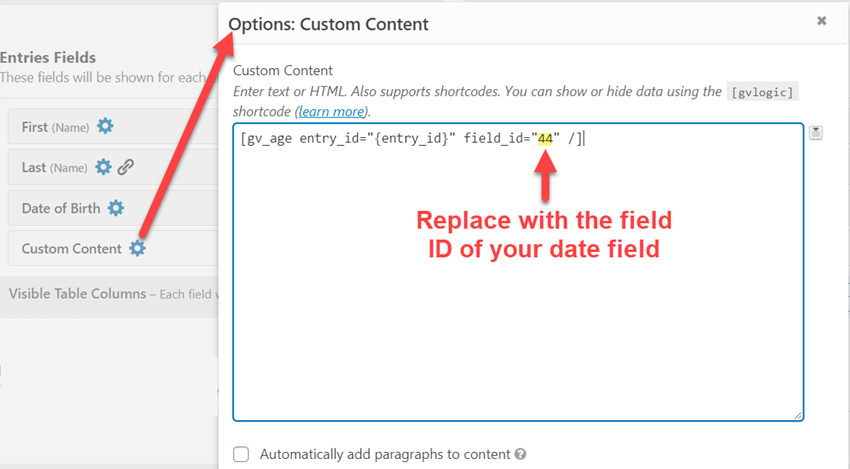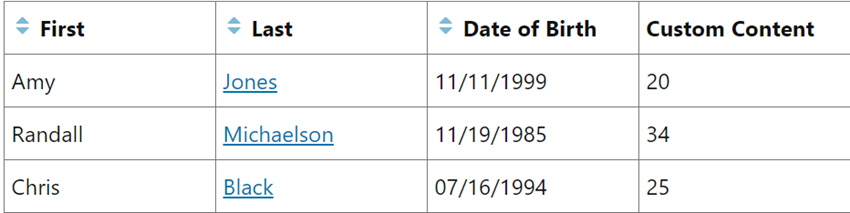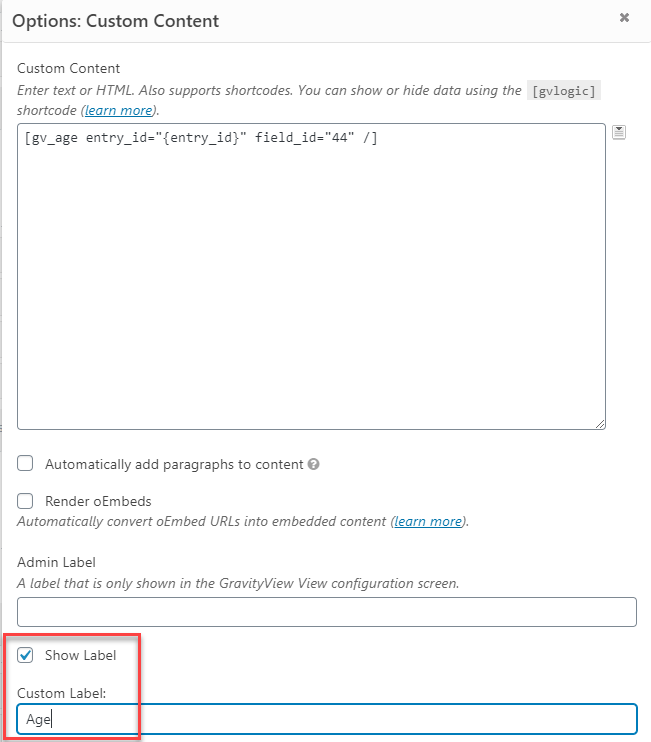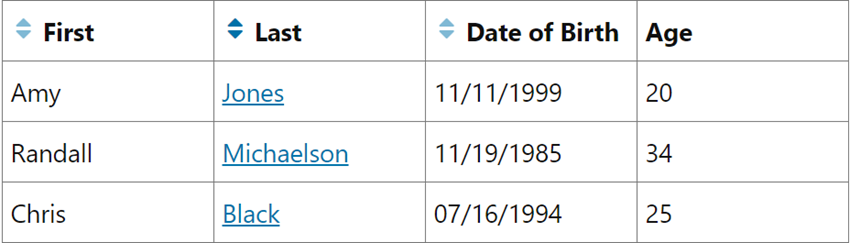# Calculating a person's age based on a date field

Ever wanted to calculate someone else's age based on a date field? That's possible using the `[gv_age]` shortcode.

### Shortcode Parameters

The `[gv_age]` shortcode requires two parameters to calculate age.

• `entry_id` - The ID of the entry for the age calculation. Can use the `{entry_id}` Merge Tag or a numeric entry ID.
• `field_id` - The ID of the date field where date of birth is stored.

### Example

This shortcode will calculate the ages for all entries. Date of birth is stored in a date field with an ID of 44:

```[gv_age entry_id="{entry_id}" field_id="44" /]
```

Adding the `[gv_age]` shortcode to a Custom Content field:The output:Now that we have the age displayed, we can enter a label for the Custom Content field:And voilá:You'll need this code to enable the `[gv_age]` shortcode:

```add_shortcode( 'gv_age', 'gv_calculate_age' );

function gv_calculate_age( \$atts ) {

\$defaults = array(
'field_id'    => '',
'entry_id'    => '',
'format'      => '%y',
'hide_errors' => ''
);

\$atts = shortcode_atts( \$defaults, \$atts, 'gv_age' );

\$entry = GFAPI::get_entry( \$atts['entry_id'] );

if ( ! \$entry || is_wp_error( \$entry ) ) {
}

if ( empty( \$entry[ \$atts['field_id'] ] ) ) {
return empty( \$atts['hide_errors'] ) ? 'Error: Field value not specified.' : '';
}

\$from = new DateTime( \$entry[ \$atts['field_id'] ] ); // Birth date
\$to   = new DateTime( 'now' );
\$interval = \$from->diff( \$to );

return \$interval->format( \$atts['format'] ); // Default format is years ('%y')
}
```